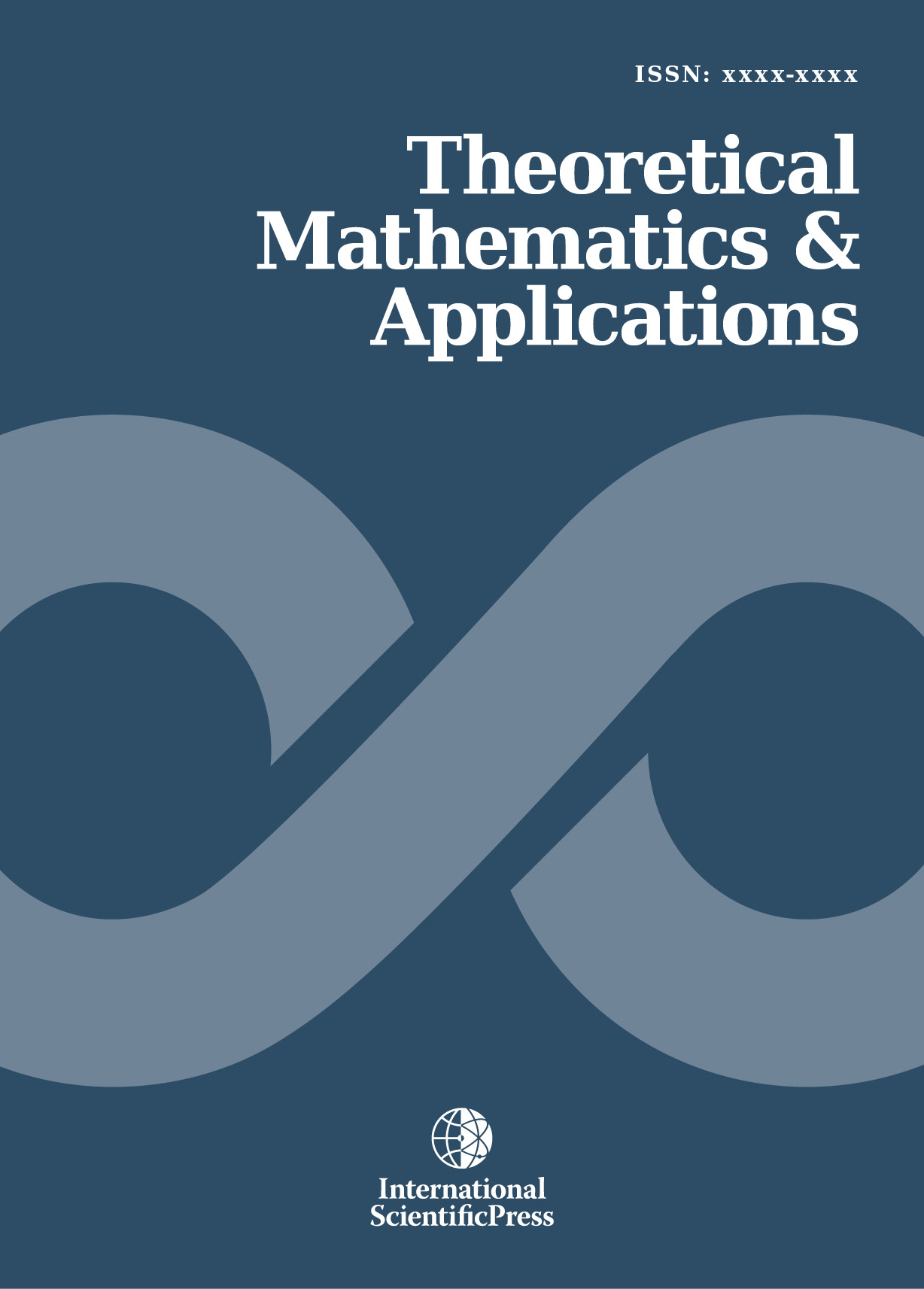# Theoretical Mathematics & Applications

#### Using homotopy analysis method for solving Volterra integral equations of the second kind

•[ Download ]
• Times downloaded: 1060
• Abstract

This paper employs an analytical method, where linear and nonlinear integral equations are solve using the homotopy analysis method. The Volterra integral equations of the second kind are considered by this method. Here an infinite solution series which converges to the exact solution of considered equations are realized. In this method one is allowed to choose an initial guess and iteratively deforms the considered equations with an initial guess to obtain the exact solution.ISSN: 1792-9687 (Print)
1792-9709 (Online)